Next: Restrictor, Wall Orifice Up: Fluid Section Types: Gases Previous: Restrictor, Contraction   Contents

### Restrictor, Bend

Properties: adiabatic, not isentropic, symmetric, inlet based restrictor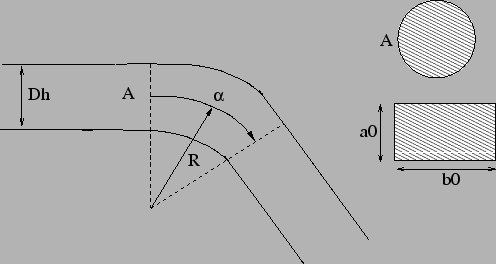The geometry of a bend is shown in Figure 99. There are three types: TYPE = RESTRICTOR BEND IDEL CIRC, TYPE = RESTRICTOR BEND IDEL RECT, both with loss coefficients according to  and TYPE = RESTRICTOR BEND MILLER with coefficients taken from . In the first and last type the bend is described by the following constants (to be specified in that order on the line beneath the *FLUID SECTION, TYPE = RESTRICTOR BEND IDEL CIRC or TYPE = RESTRICTOR BEND MILLER card):

• cross section before the bend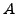.
• cross section after the bend.
• hydraulic diameter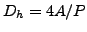, where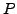is the perimeter of the cross section.
• radius of the bend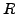.
• bend angle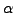(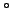).
• oil mass flow in the restrictor (only if the OIL parameter is used to define the kind of oil in the *FLUID SECTION card)
• not used (internally: oil material number)

They apply to circular cross sections. For rectangular cross sections the constants are as follows (to be specified in that order on the line beneath the *FLUID SECTION, TYPE=RESTRICTOR BEND IDEL RECT card):

• cross section before the bend.
• cross section after the bend.
• hydraulic diameter, whereis the perimeter of the cross section.
• radius of the bend.
• bend angle.
• height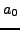.
• width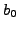.
• oil mass flow in the restrictor (only if the OIL parameter is used to define the kind of oil in the *FLUID SECTION card)
• not used (internally: oil material number)

The loss coefficients are those published by Idelchik  and Miller .

By specifying the parameter LIQUID on the *FLUID SECTION card the loss is calculated for liquids. In the absence of this parameter, compressible losses are calculated.

Example files: restrictor, restrictor-oil.Next: Restrictor, Wall Orifice Up: Fluid Section Types: Gases Previous: Restrictor, Contraction   Contents
guido dhondt 2018-12-15